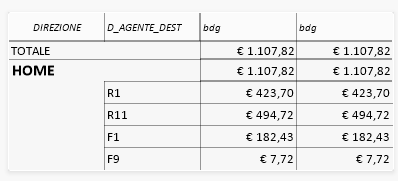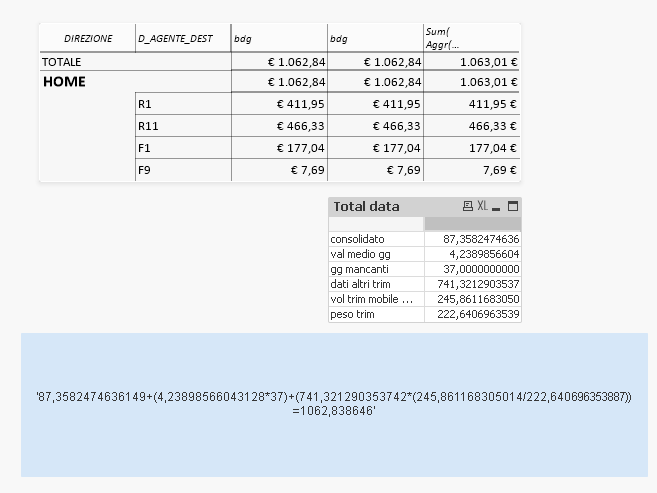QlikView Layout & Visualizations

Discussion Board for collaboration on QlikView Layout & Visualizations.

Announcements
Contributor

Pivot total value different from sum of rows value

Hi, I'm not very experienced in QlikView and I need help in this pivot tableTotal value € 1.107,82 is different from from sum of rows value

€ 423,70+

€ 494,72+

€ 182,43+

€ 7,72=

€ 1.108,58

and i don't understand why

Can someone help me?

thanks

Tags (2)
6 RepliesMVP

Re: Pivot total value different from sum of rows value

The partial sum is evaluated in total context, if you need to add up the sum of rows in a pivot table, use advanced aggregation:

=Sum ( Aggr(

YourExpression

, YourTableDimension(s)

))

Contributor

Re: Pivot total value different from sum of rows value

Thanks for the reply but there is something that I do not understand

If I try to do total counts of data without dimension(see table Total data) I get a value(1062.84) different from the one obtained using AGGR (1.063,01)And I think the correct value is 1062.84

Thanks again

Contributor

Re: Pivot total value different from sum of rows value

this is likely because your transaction can map to more than one dimension

e.g. certain transaction may both associate to R1 and R11, so it is counted in both rows

Contributor III

Re: Pivot total value different from sum of rows value

I think the solution Stefan has proposed is the correct one (i.e. it provides the correct totals, which is 1063.01 not 1062.84)

I believe the issue is both with your data and with your expressions

You have a "D_AGENTE_DEST" (F9) that is missing "W_TOT_IMP_RIGA_NETNET" for a few months

The implication of this is clear when you divide by a number that you calculate with a series of if statements; look at the expression "dati altri trim" in the "Total Data" table. You are dividing by the following expression:

if(sum(IF(ANNO_DOC = (vCurrentYear-5)  AND MAKEDATE((vCurrentYear-5) , 1, 1) >= MakeDate(2009, 1, 1) AND 2 = Ceil(MESE_DOC/3), W_TOT_IMP_RIGA_NETNET, 0)) <> 0, 1, 0) +

if(sum(IF(ANNO_DOC = (vCurrentYear-4)  AND MAKEDATE((vCurrentYear-4) , 1, 1) >= MakeDate(2009, 1, 1) AND 2 = Ceil(MESE_DOC/3), W_TOT_IMP_RIGA_NETNET, 0)) <> 0, 1, 0) +

if(sum(IF(ANNO_DOC = (vCurrentYear-3)  AND MAKEDATE((vCurrentYear-3) , 1, 1) >= MakeDate(2009, 1, 1) AND 2 = Ceil(MESE_DOC/3), W_TOT_IMP_RIGA_NETNET, 0)) <> 0, 1, 0) +

if(sum(IF(ANNO_DOC = (vCurrentYear-2)  AND MAKEDATE((vCurrentYear-2) , 1, 1) >= MakeDate(2009, 1, 1) AND 2 = Ceil(MESE_DOC/3), W_TOT_IMP_RIGA_NETNET, 0)) <> 0, 1, 0) +

if(sum(IF(ANNO_DOC = (vCurrentYear-1)  AND MAKEDATE((vCurrentYear-1) , 1, 1) >= MakeDate(2009, 1, 1) AND 2 = Ceil(MESE_DOC/3), W_TOT_IMP_RIGA_NETNET, 0)) <> 0, 1, 0)

Now, when you activate the dimension in your table, the expressions (and not the totals) are calculated correctly. The expressions above return the correct values for all "D_AGENTE_DEST"; for F9 it correctly returns 3 while it returns 5 for all other "D_AGENTE_DEST". When QlikView evaluates the totals for the expression, the above will return 5 across the whole data set (i.e. every if statement returns true because, across the whole dataset, there are no gaps of "W_TOT_IMP_RIGA_NETNET" in any month; again, this happens because the "D_AGENTE_DEST" dimension is ignored when calculating the totals). Which is incorrect because we know there is "D_AGENTE_DEST" whose result is 3 so the above should return something lower that 5.

So, the solution, as stated by Stefan, is to introduce the aggregation; the total you were calculating before in your text box (1062.84) was actually incorrect

Hope it helps

Contributor

Re: Pivot total value different from sum of rows value

Thanks for the reply
I have just one doubt:

If I create pivot tables with different dimensions, I have to change the list of dimensions present in the aggr function for each pivot table? if I do so I will have on each pivot table total values different from the others?

Thanks again

Contributor III

Re: Pivot total value different from sum of rows value

"I have to change the list of dimensions present in the aggr function for each pivot table?" - I believe so; you would need to list the dimensions used

"if I do so I will have on each pivot table total values different from the others?" - the totals should be the same but your expressions are quite complex so you'll need to understand what extra dimensions you are bringing in and what impact it will have on the calculations. Take for example the division discussed before; that relies on the data being presented without "ANNO_DOC" or "MESE_DOC" as dimensions otherwise the denominator won't be calculated correctly. Probably you would need to test it to ensure you are getting the desired results